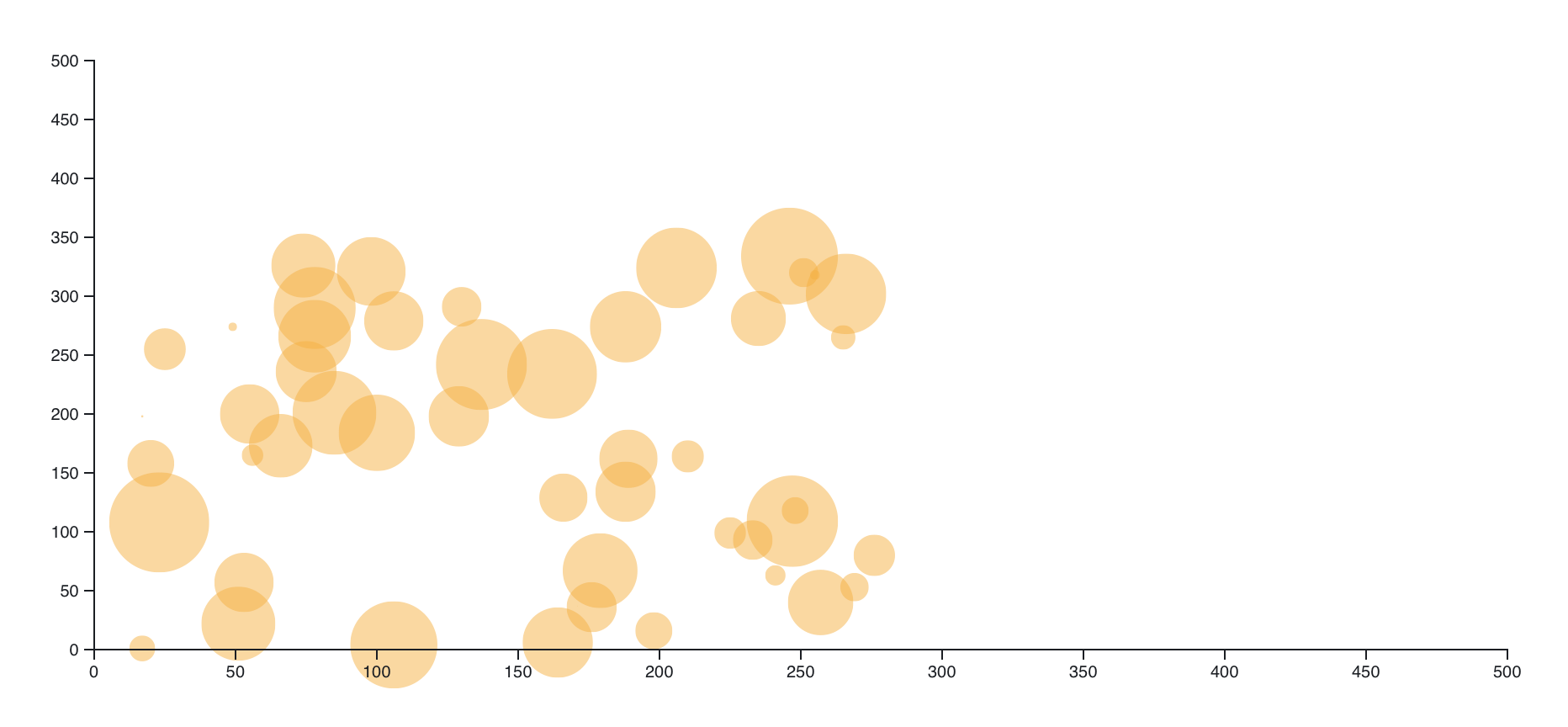# Selection

Selection is the assignment and manipulation of elements in the construction of a chart. In D3 there are a range of methods that are used to add and filter elements in order to allocate specific data and apply modifications. The most widely used among these are the `.select()`, `.selectAll()` and `.append()` methods.

The following sections are a brief introduction to the role and implementation of selections in D3. Entries that cover these methods in more depth are linked at the bottom.

## Syntax

``````svg.selectAll("element")     // A selection method is called on an object
.data("source")           // additional methods are chained to the declaration
.join("element")
.attr("x", d => d)   // The element "selected" is now defined via attributes
.attr("y", d => d)
...
``````

The pseudo code above represents a pattern commonly found in D3 for binding data and graphic elements, it is the `selectAll()/data()/join()/attr()` pattern. A selection begins with an initialization, `.selectAll("element")`, and then the elements that will populate this structure/allocation are defined in turn through the chained methods that follow.

## Example

A scatterplot is one of the simplest charts, it is a representation of data in 2-D space where each element is located based on an associated x-y coordinate value. Generally, each element is marked with a point, circle, or some other shape.

For this example, the data will consist of 50 points generated with the aid of the JavaScript `Math.random()` method. The set is constructed as follows:

```let dataset = [];let totalPoints = 50;let rangeX = Math.random() * 500;let rangeY = Math.random() * 500;
for (let i = 0; i < totalPoints; i++) {  let newX = Math.floor(Math.random() * rangeX);  let newY = Math.floor(Math.random() * rangeY);  dataset.push([newX, newY]);}
```

The dataset constructed above is incorporated into the D3 chart definition using the pattern highlighted above:

```svg.selectAll("circle")          // Declaring the circles that will represent each pt   .data(dataset)                // Calling the dataset   .join("circle") .             // The circle that will match with each data pt     .attr("cx", d => x(d))   // is added and defined     .attr("cy", d => y(d))     .attr("r", d => Math.random(1,10) * 30)     .attr("fill", "#f5b042")     .attr("opacity", .5);
```

Note: Select statements can serve two functions: the declaration of a new container, as well an assignment to an existing object (e.g., referencing an entity by calling it’s ID or Class).

In this selection the `.selectAll()` method is chained to the `svg` container, and the argument `"circle"` is given. Then:

• The data previously generated is introduced via the `.data()` method.
• The element that matches with each of the data points provided is initialized with `.join()`.
• Attributes that define the element are chained together to compose the definition of `"circle"`.
• There are five characteristics defined that articulate each point. A location is provided through arrow functions that return either the x or y value translated by the associated scale. The `math.Random()` method is used again to generate radius values. And finally the color and opacity of the elements is set.

The entire chart definition is described by the following code:

```const marginTop = 20;const marginRight = 20;const marginBottom = 30;const marginLeft = 40;const width = 900;const height = 400;const svg = d3  .create('svg')  .attr('viewBox', [0, 0, width, height])  .attr('width', width)  .attr('height', height)  .attr('style', 'max-width: 100%; height: auto;');
const x = d3  .scaleLinear()  .domain([0, 500])  .range([marginLeft, width - marginRight]);
const y = d3  .scaleLinear()  .domain([0, 500])  .range([height - marginBottom, marginTop]);
svg  .selectAll('circle')  .data(dataset)  .join('circle')  .attr('cx', (d) => x(d))  .attr('cy', (d) => y(d))  .attr('r', (d) => Math.random(1, 10) * 30)  .attr('fill', '#f5b042')  .attr('opacity', 0.5);
svg  .append('g')  .attr('transform', `translate(0,\${height - marginBottom})`)  .call(d3.axisBottom(x));
svg  .append('g')  .attr('transform', `translate(\${marginLeft},0)`)  .call(d3.axisLeft(y));
container.append(svg.node());
```

Note: For details on how to implement a D3 chart see Observable.

The resulting chart will look similar to: